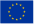next
previous
itemsFigure

# Emissions of air pollutants by sector in 2005, EU-27

Figure Created 12 Nov 2009 Published 12 Nov 2009 Last modified 29 Nov 2012
Topics:
The graph above shows the emissions of ozone precursors (methane CH4; carbon monoxide CO; nonmethane volatile organic compounds NMVOCs; and nitrogen oxides NOX) each weighted by a factor prior to aggregation to represent their respective tropospheric ozone formation potential (TOFP)

The TOFP factors are as follows: NOX 1.22, NMVOC 1, CO 0.11 and CH4 0.014 (de Leeuw, 2002). Results are expressed in NMVOC equivalents (kilotonnes kt). Data not available: for Iceland (emissions of CO, NMVOC, NOX were not reported) and Malta (CO). The figure also shows the emissions of acidifying pollutants (sulphur dioxide SO2, nitrogen oxides NOX and ammonia NH3), each weighted by an acid equivalency factor prior to aggregation to represent their respective acidification potentials. The acid equivalency factors are given by: w (SO2) = 2/64 acid eq/g = 31.25 acid eq/kg, w (NOX) = 1/46 acid eq/g = 21.74 acid eq/kg and w (NH3) = 1/17 acid eq/g = 58.82 acid eq/kg. The graph shows the emissions of primary PM10 particles (particulate matter with a diameter of 10 ¼m or less, emitted directly into the atmosphere).